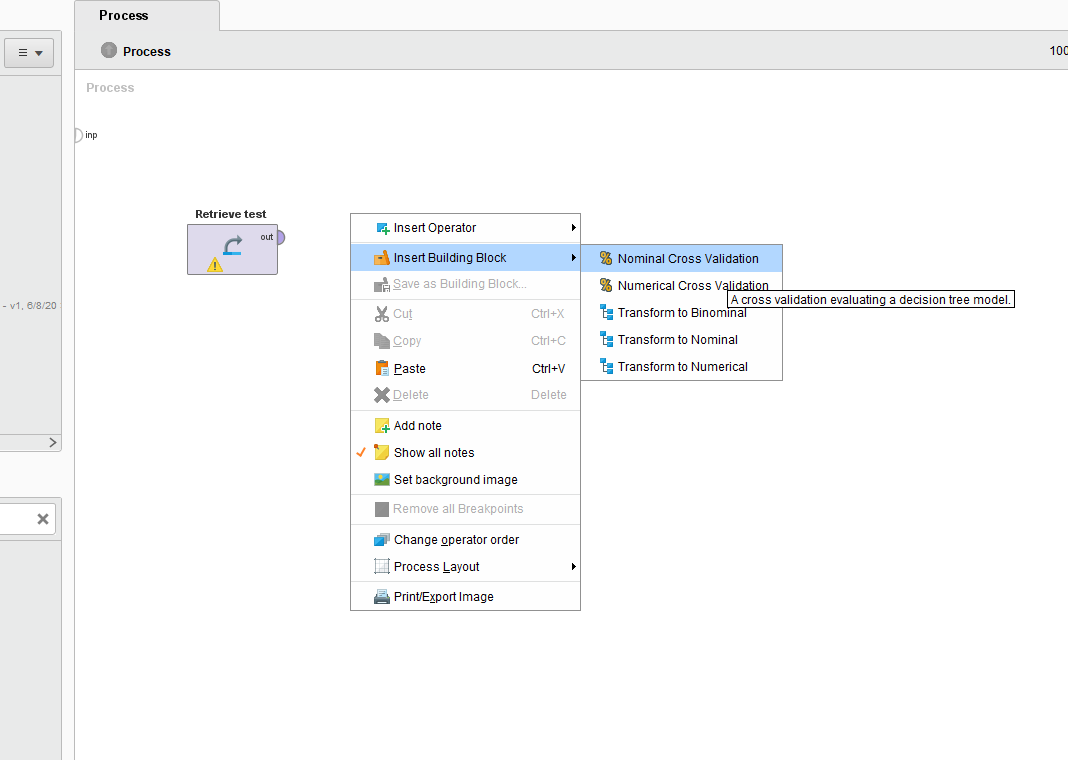# Insert determine influence factors

Member Posts: 1Newbie
Hi friends, I try to identify key sensors (or values)  that would probably be the dominant predictors of any sort of maintenance on my machines ( or dataset). I know that there is a building block that’s useful. This is to set of operators that have already built-in RapidMiner for this purpose. But in my Rapidminer(Version 9.5 ) I can t see "determine influence factor" in the insert building block. Can you help me?
Tagged:

• Member Posts: 102Unicorn
@sepi

I think you are talking about the building block know as determine influence factor, in one of the Rapidminer training videos. It is not stored as default in Rapidminer and is constructed by the rapidminer team. Please check out the below XML which you can use to generate the sub-process used for saving the block.

```<?xml version="1.0" encoding="UTF-8"?><process version="9.7.000">
<context>
<input/>
<output/>
<macros/>
</context>
<operator activated="true" class="process" compatibility="9.7.000" expanded="true" name="Process">
<parameter key="logverbosity" value="init"/>
<parameter key="random_seed" value="2001"/>
<parameter key="send_mail" value="never"/>
<parameter key="process_duration_for_mail" value="30"/>
<parameter key="encoding" value="SYSTEM"/>
<process expanded="true">
<operator activated="true" class="subprocess" compatibility="9.7.000" expanded="true" height="82" name="Determine Influence Factors" width="90" x="648" y="238">
<process expanded="true">
<operator activated="true" class="weight_by_correlation" compatibility="9.7.000" expanded="true" height="82" name="Weight by Correlation" width="90" x="45" y="34">
<parameter key="normalize_weights" value="false"/>
<parameter key="sort_weights" value="true"/>
<parameter key="sort_direction" value="ascending"/>
<parameter key="squared_correlation" value="false"/>
</operator>
<operator activated="true" class="weights_to_data" compatibility="9.7.000" expanded="true" height="68" name="Weights to Data" width="90" x="179" y="34"/>
<operator activated="true" class="generate_attributes" compatibility="6.4.000" expanded="true" height="82" name="Generate Attributes (2)" width="90" x="313" y="34">
<list key="function_descriptions">
<parameter key="Method" value="&quot;Correlation&quot;"/>
</list>
<parameter key="keep_all" value="true"/>
</operator>
<operator activated="true" class="weight_by_gini_index" compatibility="9.7.000" expanded="true" height="82" name="Weight by Gini Index" width="90" x="45" y="120">
<parameter key="normalize_weights" value="false"/>
<parameter key="sort_weights" value="true"/>
<parameter key="sort_direction" value="ascending"/>
</operator>
<operator activated="true" class="weight_by_information_gain" compatibility="9.7.000" expanded="true" height="82" name="Weight by Information Gain" width="90" x="45" y="210">
<parameter key="normalize_weights" value="false"/>
<parameter key="sort_weights" value="true"/>
<parameter key="sort_direction" value="ascending"/>
</operator>
<operator activated="true" class="weight_by_information_gain_ratio" compatibility="9.7.000" expanded="true" height="82" name="Weight by Information Gain Ratio" width="90" x="45" y="300">
<parameter key="normalize_weights" value="false"/>
<parameter key="sort_weights" value="true"/>
<parameter key="sort_direction" value="ascending"/>
</operator>
<operator activated="true" class="weights_to_data" compatibility="9.7.000" expanded="true" height="68" name="Weights to Data (2)" width="90" x="179" y="120"/>
<operator activated="true" class="generate_attributes" compatibility="6.4.000" expanded="true" height="82" name="Generate Attributes (3)" width="90" x="313" y="120">
<list key="function_descriptions">
<parameter key="Method" value="&quot;Gini&quot;"/>
</list>
<parameter key="keep_all" value="true"/>
</operator>
<operator activated="true" class="weights_to_data" compatibility="9.7.000" expanded="true" height="68" name="Weights to Data (3)" width="90" x="179" y="210"/>
<operator activated="true" class="generate_attributes" compatibility="6.4.000" expanded="true" height="82" name="Generate Attributes (4)" width="90" x="313" y="210">
<list key="function_descriptions">
<parameter key="Method" value="&quot;InfoGain&quot;"/>
</list>
<parameter key="keep_all" value="true"/>
</operator>
<operator activated="true" class="weights_to_data" compatibility="9.7.000" expanded="true" height="68" name="Weights to Data (4)" width="90" x="179" y="300"/>
<operator activated="true" class="generate_attributes" compatibility="6.4.000" expanded="true" height="82" name="Generate Attributes (5)" width="90" x="313" y="300">
<list key="function_descriptions">
<parameter key="Method" value="&quot;InfoGainRatio&quot;"/>
</list>
<parameter key="keep_all" value="true"/>
</operator>
<operator activated="true" class="append" compatibility="9.7.000" expanded="true" height="145" name="Append" width="90" x="447" y="34">
<parameter key="datamanagement" value="double_array"/>
<parameter key="data_management" value="auto"/>
<parameter key="merge_type" value="all"/>
</operator>
<operator activated="true" class="pivot" compatibility="9.7.000" expanded="true" height="82" name="Pivot" width="90" x="581" y="34">
<parameter key="group_attribute" value="Attribute"/>
<parameter key="index_attribute" value="Method"/>
<parameter key="consider_weights" value="true"/>
<parameter key="weight_aggregation" value="sum"/>
<parameter key="skip_constant_attributes" value="true"/>
<parameter key="datamanagement" value="double_array"/>
<parameter key="data_management" value="auto"/>
</operator>
<operator activated="true" class="generate_aggregation" compatibility="6.5.002" expanded="true" height="82" name="Generate Aggregation" width="90" x="715" y="34">
<parameter key="attribute_name" value="Importance"/>
<parameter key="attribute_filter_type" value="value_type"/>
<parameter key="attribute" value=""/>
<parameter key="attributes" value=""/>
<parameter key="use_except_expression" value="false"/>
<parameter key="value_type" value="numeric"/>
<parameter key="use_value_type_exception" value="false"/>
<parameter key="except_value_type" value="time"/>
<parameter key="block_type" value="attribute_block"/>
<parameter key="use_block_type_exception" value="false"/>
<parameter key="except_block_type" value="value_matrix_row_start"/>
<parameter key="invert_selection" value="false"/>
<parameter key="include_special_attributes" value="false"/>
<parameter key="aggregation_function" value="average"/>
<parameter key="concatenation_separator" value="|"/>
<parameter key="keep_all" value="true"/>
<parameter key="ignore_missings" value="true"/>
<parameter key="ignore_missing_attributes" value="false"/>
</operator>
<operator activated="true" class="normalize" compatibility="7.5.003" expanded="true" height="103" name="Normalize" width="90" x="849" y="34">
<parameter key="return_preprocessing_model" value="false"/>
<parameter key="create_view" value="false"/>
<parameter key="attribute_filter_type" value="single"/>
<parameter key="attribute" value="Importance"/>
<parameter key="attributes" value=""/>
<parameter key="use_except_expression" value="false"/>
<parameter key="value_type" value="numeric"/>
<parameter key="use_value_type_exception" value="false"/>
<parameter key="except_value_type" value="real"/>
<parameter key="block_type" value="value_series"/>
<parameter key="use_block_type_exception" value="false"/>
<parameter key="except_block_type" value="value_series_end"/>
<parameter key="invert_selection" value="false"/>
<parameter key="include_special_attributes" value="false"/>
<parameter key="method" value="range transformation"/>
<parameter key="min" value="0.0"/>
<parameter key="max" value="1.0"/>
<parameter key="allow_negative_values" value="false"/>
</operator>
<operator activated="true" class="sort" compatibility="9.7.000" expanded="true" height="82" name="Sort (2)" width="90" x="581" y="120">
<parameter key="attribute_name" value="Importance"/>
<parameter key="sorting_direction" value="decreasing"/>
</operator>
<operator activated="true" class="order_attributes" compatibility="9.7.000" expanded="true" height="82" name="Reorder Attributes (3)" width="90" x="715" y="120">
<parameter key="sort_mode" value="user specified"/>
<parameter key="attribute_ordering" value="Attribute|Importance"/>
<parameter key="use_regular_expressions" value="false"/>
<parameter key="handle_unmatched" value="remove"/>
<parameter key="sort_direction" value="ascending"/>
</operator>
<connect from_port="in 1" to_op="Weight by Correlation" to_port="example set"/>
<connect from_op="Weight by Correlation" from_port="weights" to_op="Weights to Data" to_port="attribute weights"/>
<connect from_op="Weight by Correlation" from_port="example set" to_op="Weight by Gini Index" to_port="example set"/>
<connect from_op="Weights to Data" from_port="example set" to_op="Generate Attributes (2)" to_port="example set input"/>
<connect from_op="Generate Attributes (2)" from_port="example set output" to_op="Append" to_port="example set 1"/>
<connect from_op="Weight by Gini Index" from_port="weights" to_op="Weights to Data (2)" to_port="attribute weights"/>
<connect from_op="Weight by Gini Index" from_port="example set" to_op="Weight by Information Gain" to_port="example set"/>
<connect from_op="Weight by Information Gain" from_port="weights" to_op="Weights to Data (3)" to_port="attribute weights"/>
<connect from_op="Weight by Information Gain" from_port="example set" to_op="Weight by Information Gain Ratio" to_port="example set"/>
<connect from_op="Weight by Information Gain Ratio" from_port="weights" to_op="Weights to Data (4)" to_port="attribute weights"/>
<connect from_op="Weights to Data (2)" from_port="example set" to_op="Generate Attributes (3)" to_port="example set input"/>
<connect from_op="Generate Attributes (3)" from_port="example set output" to_op="Append" to_port="example set 2"/>
<connect from_op="Weights to Data (3)" from_port="example set" to_op="Generate Attributes (4)" to_port="example set input"/>
<connect from_op="Generate Attributes (4)" from_port="example set output" to_op="Append" to_port="example set 3"/>
<connect from_op="Weights to Data (4)" from_port="example set" to_op="Generate Attributes (5)" to_port="example set input"/>
<connect from_op="Generate Attributes (5)" from_port="example set output" to_op="Append" to_port="example set 4"/>
<connect from_op="Append" from_port="merged set" to_op="Pivot" to_port="example set input"/>
<connect from_op="Pivot" from_port="example set output" to_op="Generate Aggregation" to_port="example set input"/>
<connect from_op="Generate Aggregation" from_port="example set output" to_op="Normalize" to_port="example set input"/>
<connect from_op="Normalize" from_port="example set output" to_op="Sort (2)" to_port="example set input"/>
<connect from_op="Sort (2)" from_port="example set output" to_op="Reorder Attributes (3)" to_port="example set input"/>
<connect from_op="Reorder Attributes (3)" from_port="example set output" to_port="out 1"/>
<portSpacing port="source_in 1" spacing="0"/>
<portSpacing port="source_in 2" spacing="0"/>
<portSpacing port="sink_out 1" spacing="0"/>
<portSpacing port="sink_out 2" spacing="0"/>
</process>
<description align="center" color="transparent" colored="false" width="126">Determines which sensors are the most important for the prediction based upon multiple weighting algorithms.</description>
</operator>
<portSpacing port="source_input 1" spacing="0"/>
<portSpacing port="sink_result 1" spacing="0"/>
</process>
</operator>
</process>
```

Best,
Harshit